★ APPLICATIONS ★ DIVERS ★ Discourse II ★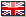Discourse II|Amstrad Action)Applications Divers

#### h = ax - by

where x and y are natural numbers.

One reason why this property is so interesting is that equations of this form were extensively studied by Diophantus (ca. 180 AD), probably the best, ancient algebraist, and are today often referred to as Diophantine Equations.

The only known woman mathematician of the ancient Alexandrian school, Hypatia, recognised this (alas, she was battered to death by Christian fanatics in about 415 AD).

Diophantus was the first to employ signs (for positive and negative) systematically and he also used symbols for unknowns, powers and so on He solved linear, quadratic and at least one cubic equation.

In some of his methods he effectively used the device of approximating to limits, a method eventually systematized by Sir Issac Newton in the 1670's as Calculus.

Using his limit technique, Diophantus attempted to divide 13 into two square numbers, each of which is to be greater than 6. He arrived at squares with sides of 258/101 and 257/101.

Clearly, in all these matters, the knowledge as to whether any given number is prime (that is. divisible by only itself and 1) or not is of utmost importance. If any number is not divisible by any prime number in the range 2 up to its square root then that number itself must be prime. The process of checking the divisibility

of a number by the primes is very laborious and many mathematicians have developed methods to reduce this labour.

Gauss (1777-1855) and Pierre Format (1601-1665) were quite successful and Fermat's method, which is extremely simple in principle, is explained below.

Let P be the number we wish to test and

let n be the lowest number such that n> P. All we have to do now is form the series of numbers

#### n2 - P , ( n + 1) 2 - P , ( n + 2 ) 2 - P ...

If a number is reached which is a perfect square then we have

#### x2 - p = y2 and thus P = X2 - Y2 = (X = y);

If P is prime then the solution we reach gives x + y-Pand x - y = 1.

The difference between each term of the series is 2n = m wherein is 1,3.5.... as the algorithm progresses.

An example illustrates this best. Let P = 9271. This lies between 962 and 972 , so  that n = 97. Our series is thus

#### n2 - P    972 - 9271 = 9409 - 9271 = 138+ 2n + 1                          = 333+ 2n + 3                          = 530+ 2n + 5                          = 729+ 2n + 7                          = 930

but 729 is the perfect square of 27, thus we have x2 - 9271 = 729. Therefore x=100 and, from above , P = (x + y)(x - y) , 9271 = 123 x 73.

The program below docs exactly this. Line 90 takes on the number to be factorised, which should be greater than 1000, and as an integer, less than 32767.

Line 110 calculates the starting value of n. e is the integer value to test if the value of the series member r is a perfect square, which is done in the gosub 300 routine. If r is a perfect square the subroutine returns f = 1 and drops to Line 250, when the Factors are calculated and printed in Line 280.

AA

 ★ PUBLISHER: AMSTRAD ACTION★ YEAR: 1989★ CONFIG: 64K + AMSDOS★ LANGUAGE:★ LICENCE: LISTING★ AUTHOR: JAMES WILSON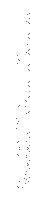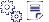Type-in/Listing:» Discourse  2    LISTINGDATE: 2014-03-24DL: 11 foisTYPE: PDFSIZE: 93KoNOTE: 1 page/PDFlib v1.6Je participe au site:» Newfile(s) upload/Envoye de fichier(s)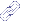Lien(s):» Applications » RSX - Circle» Applications » Halley-Luiah (The Amstrad User)» Applications » Electron (CPC Revue)» Applications » Rsx - Programm - Tracer (CPC Amstrad International)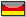» Applications » Initimpr (Tilt)» Applications » Encode/Decode